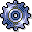Depreciation Calculation MethodsUse

The depreciation calculation method is the most important characteristic of the base method. The depreciation calculation method makes it possible to carry out the numerous different types of depreciation calculation in the system. Depending on how the depreciation calculation method is set up, the system determines which further control parameters need to be specified in the depreciation key and which do not. For example, when you choose the Stated percentage depreciation calculation method, you have to enter a percentage in the depreciation key.

The depreciation calculation methods listed below are provided in the standard system.

Features

Percentage from Useful Life / Percentage from Remaining Useful Life

There are two versions of this depreciation calculation method. In the first, the system determines a depreciation percentage rate from the total useful life; the rate remains the same for each year. The second version calculates a new percentage rate for each year based on the remaining useful life. In this version, the depreciation percentage rate rises constantly and then reaches 100% in the last year of the useful life.

Total Percentage Rate in the Tax Concession Period

This method allows you to depreciate a certain percentage rate from the depreciation base within a tax concession period. In order to calculate the current periodic depreciation, the system first determines the accumulated depreciation up to the period under examination. The period depreciation is the difference between the already existing depreciation and the total depreciation allowed. With subsequent acquisitions, the system automatically catches up depreciation from previous years in a lump sum.

Stated Percentage Rate

In contrast to a total percentage rate, here you specify the percentage rate for each fiscal year. The system uses this percentage rate for calculating depreciation for each period. For example, you can depreciate 3.5% in each of the first 12 years, then 2% a year for 20 years and 1% per year for the remaining 18 years. The total of the percentage rates over the useful life is logically always 100%, so that complete depreciation is reached by the end of the useful life.

Percentage Rate from Remaining Life + Changeover Date - Depreciation Start Date

This method is used as a changeover method (in the next phase in the depreciation key) following depreciation within the tax concession period of an investment support measure. The net book value of the asset will be depreciated over the total useful life when the tax concession period ends (that is, the actual duration of depreciation encompasses the tax concession period plus the total useful life that is entered).

Mean Value from Several Areas

When defining depreciation areas, you can establish dependencies between them by specifying a mathematical formula. This method allows you to calculate depreciation in one area based on the depreciation in another area using this mathematical formula. Using this method you can, for example, calculate the mean value from straight-line depreciation and declining balance depreciation.

Unit-of-Production Depreciation

Unit-of-production depreciation is based on the output-related use of the asset. When you specify a total expected output or a total expected number of units, and the exact output per period or exact unit of production output figure per period, the system determines the resulting depreciation for each period. You enter the output or number of units at the level of the depreciation key.

Depreciation Over Remaining Units of Production

In the same way as with the unit-of-production method of depreciation, the amount of depreciation here is dependent on output. In contrast to the unit-of-production method of depreciation, the system uses the remaining units of production and not the total units of production to determine the periodic depreciation. Depreciating using the remaining units of production ensures that, for post-capitalization, the book value reaches zero when the total output or the total units of production is reached.

Sum-of-the-Years-Digits Method

An arithmetic sequence is set up based on the total useful life. The depreciation percentage rate is proportional to the remaining useful life.

Depreciation According to the Present Value of Lease Installments

This depreciation calculation method is designed for leased assets that have been capitalized using the capital lease procedure (refer to Leased Assets). The depreciation amounts correspond here to the present value of the periodic lease installments. The interest is determined as the difference between the lease installment and the present value.

Declining-Balance Method of Depreciation According to Japanese Regulations